#### The cost of a feedback plan

Consider the cost-to-go of executing a plan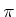from a state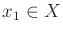. The resulting cost depends on the sequences of states that are visited, actions that are applied by the plan, and the applied nature actions. In Chapter 2 this was obtained by adding the cost terms, but now there is a dependency on nature. Both worst-case and expected-case analyses are possible, which generalize the treatment of Section 9.2 to state spaces and multiple stages.

Let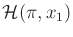denote the set of state-action-nature histories that could arise fromwhen applied using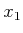as the initial state. The cost-to-go,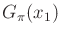, under a given planfromcan be measured using worst-case analysis as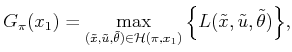(10.31)

which is the maximum cost over all possible trajectories fromunder the plan. If any of these fail to terminate in the goal, then the cost becomes infinity. In (10.31),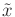,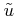, and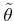are infinite histories, although their influence on the cost is expected to terminate early due to the application of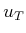.

An optimal plan using worst-case analysis is any plan for whichis minimized over all possible plans (all ways to assign actions to the states). In the case of feasible planning, there are usually numerous equivalent alternatives. Sometimes the task may be only to find a feasible plan, which means that all trajectories must reach the goal, but the cost does not need to be optimized.

Using probabilistic uncertainty, the cost of a plan can be measured using expected-case analysis as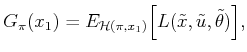(10.32)

in which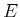denotes the mathematical expectation taken over(i.e., the plan is evaluated in terms of a weighted sum, in which each term has a weight for the probability of a state-action-nature history and its associated cost,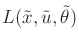). This can also be interpreted as the expected cost over trajectories from. If any of these have nonzero probability and fail to terminate in the goal, then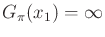. In the probabilistic setting, the task is usually to find a plan that minimizes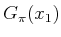.

An interesting question now emerges: Can the same plan,, be optimal from every initial state, or do we need to potentially find a different optimal plan for each initial state? Fortunately, a single plan will suffice to be optimal over all initial states. Why? This behavior was also observed in Section 8.2.1. Ifis optimal from some, then it must also be optimal from every other state that is potentially visited by executingfrom. Letdenote some visited state. Ifwas not optimal from, then a better plan would exist, and the goal could be reached fromwith lower cost. This contradicts the optimality ofbecause solutions must travel through. Let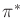denote a plan that is optimal from every initial state.

Steven M LaValle 2020-08-14